# Isotherm analysis

## Introduction​

Adsorption is a process of adhesion of atoms, ions or molecules on a surface. Desorption is the inverse process. There are two types of adsorption:

These adsorptions can be described by adsorption isotherms. If the adsorbate is a gas, its amount is plotted against its pressure, if it consists of a liquid phase solute, it is plotted as a function of the concentration. In both cases, the process occurs at a constant temperature.

There are several models to describe adsorption. For example the Langmuir adsorption Isotherm or the BET Theory.

The following assumptions are made :

• The surface has finite number of identical adsorption sites $N$.
• There can be only a single adsorbate per site ($\theta_{\text{max}} = 1$: monolayer).
• The enthalpy of adsorption ($\Delta H_{\text{abs}}$) is independent of the coverage $\theta$, we suppose no interaction between the adsorbates.

If $K_a$ is the equilibrium constant and $p_a$ the partial pressure of the gas, the coverage is given by the Langmuir isotherm:

$\theta = \frac{K_a p_a}{1+K_a p_a}, \quad \theta \in [0, 1]$
Limitations of the Langmuir model

In this model, the interactions between the adsorbed molecules are neglected and it is only a monolayer adsorption.

### BET (Brunauer–Emmett–Teller) Theory​

• Gas molecules physically adsorb on a solid in layers infinitely.
• Gas molecules only interact with adjacent layers.
• The Langmuir model can be applied to each layer.
• The enthalpy of adsorption for the first layer is constant and greater than the second (and higher).
• The enthalpy of adsorption for the second (and higher) layers is the same as the enthalpy of liquefaction.

With these assumptions, the BET equation is given as :

$\theta=\frac{cp}{(1-p/p_o)\bigl(p_o+p(c-1)\bigr)}$

Where $c$ is the BET $c$-constant, $p_o$ is the vapor pressure of the adsorptive bulk liquid phase and $\theta$ is the surface coverage.

Files can be uploaded either by drag-and-drop to the field on the left-hand-side or automatically from the instrument. The files will appear in field 2. Note that you can only upload files to samples to which you have write access.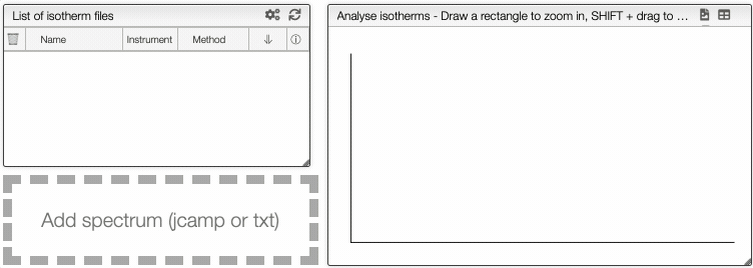Supported file types

We currently support the file types (which are also automatically detected):

• xls files produces by Belsorp instruments
• csv files produced by Belsorp instruments
• txt files produced by IGA instruments
• txt files produced by micrometrics instruments
• csv files produced by micrometrics instruments

If multiple desorption/adsorption cycles are stored in one file they will also be converted to one JCAMP file by our parsers.

If you need support for other file formats, open an issue on the GitHub repository or post a question in the user forum.

## Visualization​

This graph analysis use a dropdown menu switcher. If there are multiple experiments or columns in a file, you can select the ones that you want to plot using the dropdown selectors.

It is possible to see the dependance of one variable as a function of another.

At the top of the graph, you can see three dropdown menus.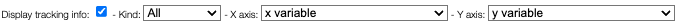The first one is the choice of either all curve or either adsorption or desorption. The two other menus are the variables that you want to show as a dependence.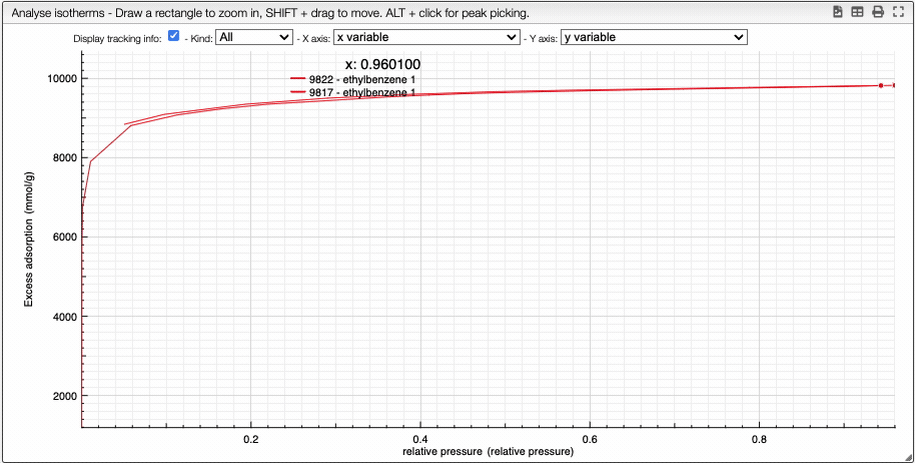You can manipulate the graph in the same way than the IR spectrum graph.

At the top of the graph, you can export the image as a svg file, you can remove the grid or even export as a pdf file.

## Compare data​

By clicking on the button Compare, you can compare multiple isotherms. You can also select which curve you want to plot and choose the color.

Visualising spectra
How to show/hide spectra.

## Visualising spectra​

If you wish to see only certain spectra, it is possible to selectively hide (or delete) them.

In order to do so, use the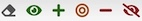buttons on the top panel of the displayed spectra list.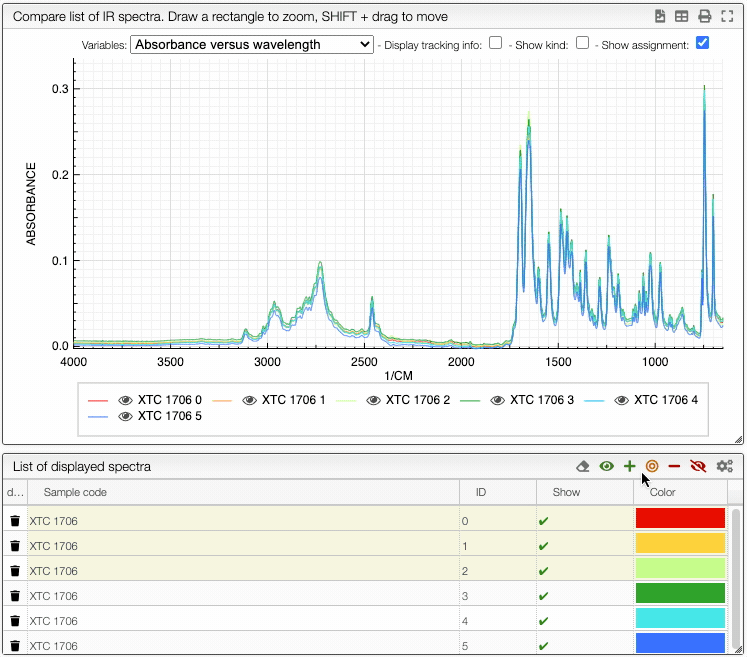You can also change the color of an individual spectrum in the displayed spectra tab with a double click.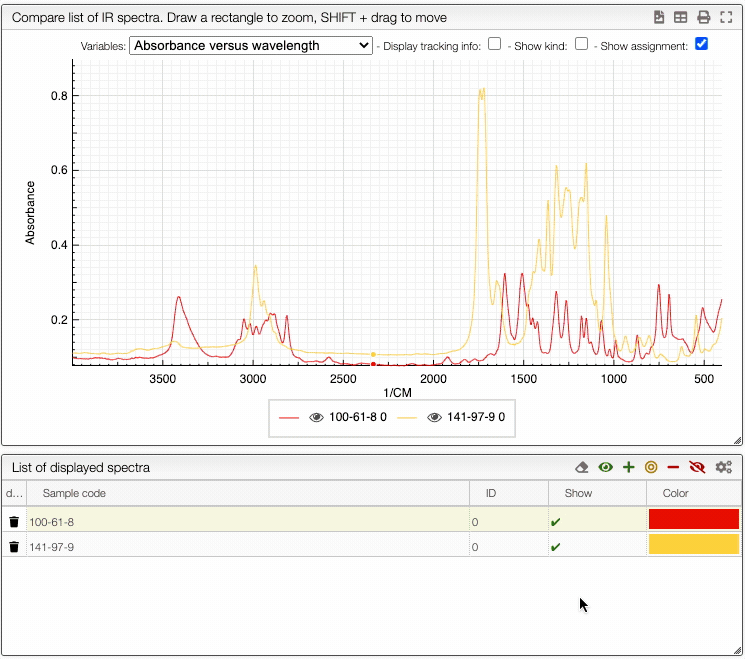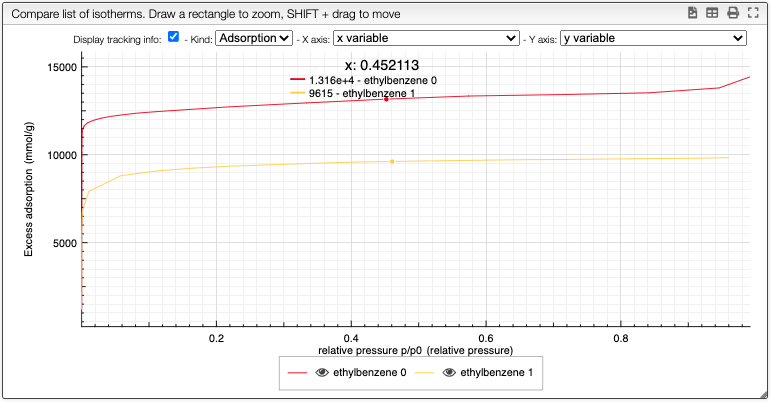note

You can still still select the adsorption/desorption as well as the multivariable dependance.

The right box can be use to hide regions of the graph or take the derivative for example.

Spectrum preprocessing
Apply mathematical tools to the spectra.

## Preprocessing​

You can apply the following modifications to the spectra to enhance the visualization. The modifications include the following:

• Center Mean : subtract the mean from every variable observation in the dataset, so that the new variable's mean is centered at 0.
• Divide by SD : divide every variable observable in the dataset by the standard deviation yields a distribution with a standard deviation equal to 1.
• Divide by max Y : divide every value by the maximum y-value shifts all the y-values between 0 and 1.
• Rescale (x to y) : rescale the graph such that the y-values fit between specified minimum and maximum values.
• Normalize (sum to n) : normalize the integral under the curve so that it sums to n.
• Multiply (value) : multiply every y-value by a scalar.
• Add (value) : add a scalar to every y-value.
• AirPLS baseline : baseline correction using adaptive iterative reweighed penalized least squares algorithm.
• Rolling average baseline :
• Iterative polynomial baseline : baseline correction using iterative polynomial fitting algorithm.
• Rolling ball baseline :
• Rolling median baseline :

A certain range of x-values can be selected to show only a part of the spectrum using Range.

Depending on the analysis, some regions should be removed using Exclusions in order to improve the visualization.